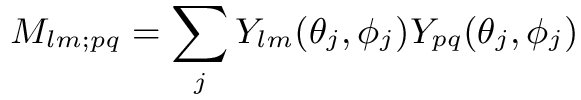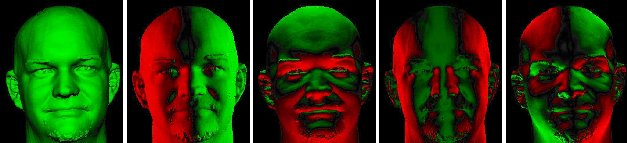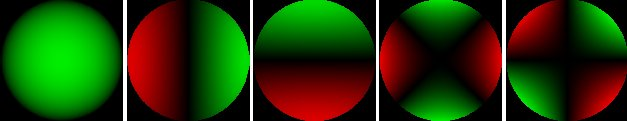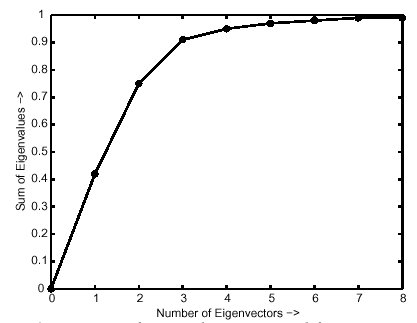## Analytic PCA construction for theoretical analysis of lighting variability in images of a Lambertian object

### Abstract

We analyze theoretically the subspace best approximating images of a convex Lambertian object taken from the same viewpoint, but under different distant illumination conditions. Since the lighting is an arbitrary function, the space of all possible images is formally infinite-dimensional. However, previous empirical work has shown that images of largely diffuse objects actually lie very close to a 5-dimensional subspace. In this paper, we analytically construct the principal component analysis for images of a convex Lambertian object, explicitly taking attached shadows into account, and find the principal eigenmodes and eigenvalues with respect to lighting variability. Our analysis makes use of an analytic formula for the irradiance in terms of spherical-harmonic coefficients of the illumination, and shows, under appropriate assumptions, that the principal components or eigenvectors are identical to the spherical harmonic basis functions evaluated at the surface normal vectors. Our main contribution is in extending these results to the single-viewpoint case, showing how the principal eigenmodes and eigenvalues are affected when only a limited subset (the upper hemisphere) of normals is available, and the spherical harmonics are no longer orthonormal over the restricted domain. Our results are very close, both qualitatively and quantitatively, to previous empirical observations and represent the first essentially complete theoretical explanation of these observations. Our analysis is also likely to be of interest in other areas of computer vision and image-based rendering. In particular, our results indicate that using complex illumination for photometric problems in computer vision is not significantly more difficult than using directional sources.

### Main Results

The main results are illustrated with the figures on the right. The major mathematical result is that the principal components and associated eigenvalues correspond to the eigenvectors of a scaled version of the orthogonality matrix , i.e. a matrix expressing the orthogonality between the spherical harmonic basis functions, when the domain of integration is restricted to the surface normals in the image. If we were considering the entire gaussian sphere of possible normal directions, this matrix would just be the scaled identity matrix. Hence, the eigenvectors or principal components would simply be the spherical harmonic modes. However, if we consider only a single image, the orthogonality matrix is no longer the identity, and the eigenvectors or principal components are linear combinations of the spherical harmonics, with a new eigenvalue spectrum. The orthogonality matrix depends on the distribution of surface normals in the image, and hence depends on object geometry. We are able to derive quantitative results for a number of cases of interest, including images of a human face and a sphere, shown on the right. The principal components and associated eigenvalue spectrum derived by our method agree very well with empirical observations for all cases of interest considered by us.

PAMI Oct 2002 paper final version in PDF (.8M)

Earlier shorter CVPR 2001 workshop on identifying objects across lighting variations paper (slightly different title) in
Gzipped Postscript (1.1M) or PDF (.6M)Orthogonality Matrix: The major mathematical result is that the principal components and associated eigenvalues correspond to the eigenvectors of a scaled version of the orthogonality matrix expressing the orthogonality between the spherical harmonic basis functions when the domain of integration is restricted to the surface normals in the image, i.e. a summation is done over the image pixels.Forms of Principal Components: The first 5 principal components for human faces computed by us agree with the labelings empirically observed by Hallinan, viz. frontal lighting, side lighting, lighting from above/below, extreme side lighting and lighting from a corner. In these principal component images, green denotes positive values while red denotes negative regions.Dimensionality of Approximating Linear Subspace: From our derivation, we conclude that 5 eigenvectors or principal components, shown here for the image of a sphere, suffice to capture over 95% of the image variance. These results agree quantitatively with empirical work.Eigenvalue Spectrum: Our results show that for human faces, the principal components lie in two groups. In a group, the eigenvalues are similar, so the principal components may exchange places. The first group, consisting of two members, corresponds to frontal and side lighting, while the next group consists of the next three principal components. The ratios of eigenvalues of the first 5 principal components are .42, .33, .16, .035, .021 with the first 5 eigenmodes accounting for 97% of the variance.
Ravi Ramamoorthi